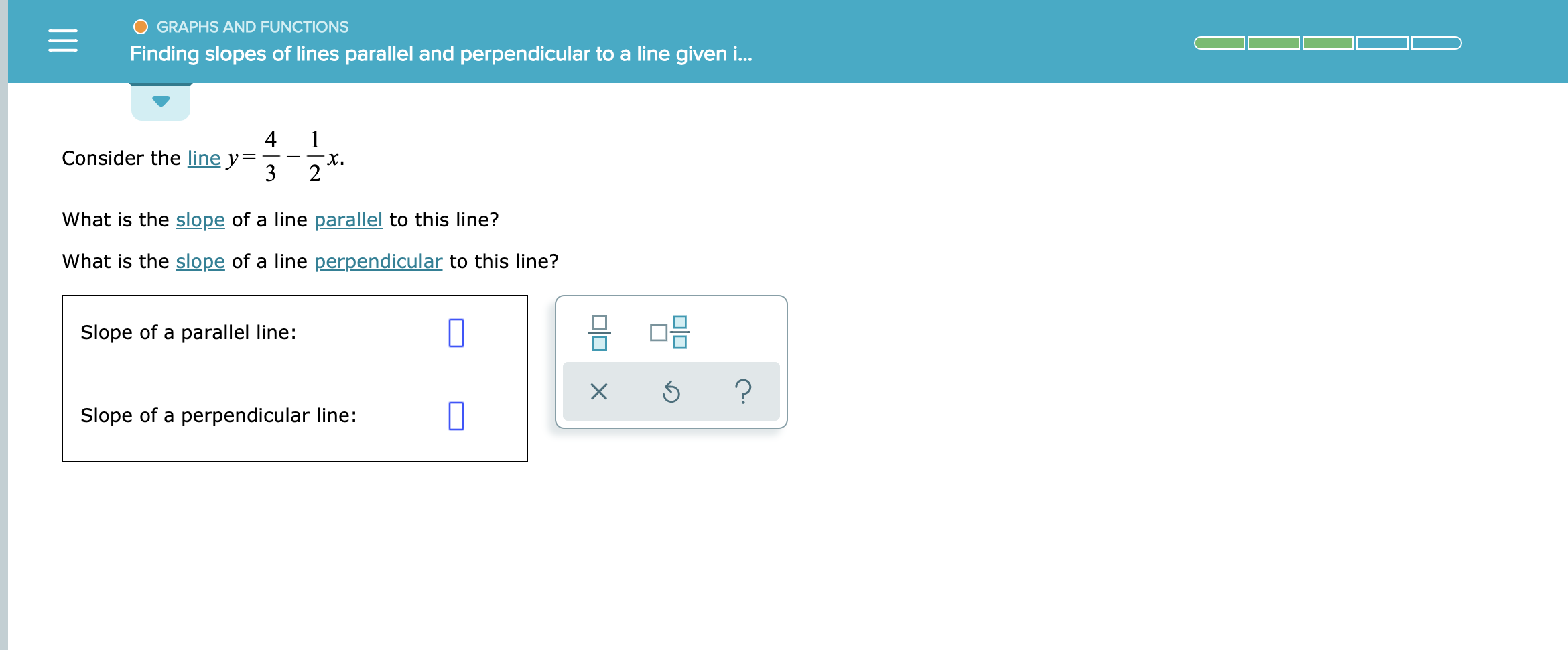# GRAPHS AND FUNCTIONSFinding slopes of lines parallel and perpendicular to a line given ..4Consider the line y312What is the slope of a line parallel to this line?What is the slope of a line perpendicular to this line?Slope of a parallel line:?Slope of a perpendicular line:X

Question
2 views

See attachedhelp_outlineImage TranscriptioncloseGRAPHS AND FUNCTIONS Finding slopes of lines parallel and perpendicular to a line given .. 4 Consider the line y 3 1 2 What is the slope of a line parallel to this line? What is the slope of a line perpendicular to this line? Slope of a parallel line: ? Slope of a perpendicular line: X fullscreen
check_circle

Step 1

Given line is y = 4/3 – 1/2 x.

Step 2

Since the parallel lines have same slope therefore the slope of given line and the line parallel to it have the same slope.

So, the required slope is given by slope of line y = 4/3 – 1/2 x

Comparing this equation with the equation of line (y = mx + c) having slope m and y-intercept equals to c.
It gives m = -1/2 and c = 4/3

Thus, the required slope is -1/2

Step 3

If two lines are perpendicular having slopes m1 and m2 then the product of their slope is equals to -1 (i.e. m1 × m2 = -1).
let m1 be the slope of given line and m2 be the slope...

### Want to see the full answer?

See Solution

#### Want to see this answer and more?

Solutions are written by subject experts who are available 24/7. Questions are typically answered within 1 hour.*

See Solution
*Response times may vary by subject and question.
Tagged in

### Calculus